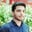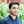Trusted answers to developer questions
Trusted Answers to Developer Questions

Related Tags

numpy
bitwise_or
communitycreator

# What is the bitwise_or() method in NumPy?Salman Yousaf

Grokking Modern System Design Interview for Engineers & Managers

Ace your System Design Interview and take your career to the next level. Learn to handle the design of applications like Netflix, Quora, Facebook, Uber, and many more in a 45-min interview. Learn the RESHADED framework for architecting web-scale applications by determining requirements, constraints, and assumptions before diving into a step-by-step design process.

### Overview

In NumPy, the bitwise_or() method is used to calculate bitwise OR between two input arrays, element-wise. It performs bitwise logical OR of underlying binary representation.

### Syntax

 numpy.bitwise_or(arr1,
arr2,
out=None,
where=True,
**kwargs)

### Parameters

It takes the following argument values.

• arr1: This is an integer and it handles boolean type arrays.
• arr2: This is an integer and it handles boolean type arrays.
• out: This is a memory location in which the result will be stored. It will be of the same dimension as arr1 or arr2. Its default value is None. If its value is None, a new array of the same dimensions will be initialised.
• where: This is the value to replace the ufunc result when the condition returns True. Otherwise, the out array will retain its original output values. Its default value is True.
• **kwargs: These are additional arguments.

### Return value

if arr1 and arr2 are two scalars, it returns a scalar value. Otherwise, it returns Ndarray type objects only.

### Example

In this code, we discuss numpy.bitwise_or() with multiple scenarios: performing OR operation between integers, Python Lists, and NumPy arrays.

# importing numpy libraryimport numpy as np# performing logical OR between 16, 18print("16 OR 18:", end=" ")print(np.bitwise_or(16, 18))# logical OR between a Python list [3,13] and 12print("[3,13] OR 12:", end=" ")print(np.bitwise_or([3,13], 12))# logical OR between two lists of same sizeprint("[9,7] OR [8,35]:", end=" ")print(np.bitwise_or([9,7], [8,35]))# performing logical OR between two numpy arraysprint("np.array([2,7,255]) OR np.array([6,12,18]):", end=" ")print(np.bitwise_or(np.array([2,7,255]), np.array([6,12,18])))

### Explanation

• Line 4–5: We perform a bitwise OR between 16 and 18, it will return 18.
• Lines 7–8: We perform a bitwise OR between a list, [3,13] and 12. Hence, it will return a list, [15,12].
• Line 10–11: It returns a bitwise OR as a list, [9 39], between two lists [9,7] and [8,35].
• Line 13–14: It returns a bitwise OR as a NumPy array, [6 15 255], between two NumPy arrays, np.array([2,7,255]) and np.array([6,12,18].

### Universal function implementation

NumPy also supports universal functions that implement C or Core Python operator, |.

# importing numpy libraryimport numpy as np# creating two numpy arraysx1 = np.array([5, 9, 128])x2 = np.array([10, 30, 255])# performing logical OR using conventional | operatorprint(x1 | x2)
• Line 4: We create a NumPy array, x1, containing [5, 9, 128].
• Line 5: We create a NumPy array, x2, containing [10, 30, 255].
• Line 7: We perform a logical OR by using a conventional | operator.

RELATED TAGS

numpy
bitwise_or
communitycreator

CONTRIBUTORSalman Yousaf

Grokking Modern System Design Interview for Engineers & Managers

Ace your System Design Interview and take your career to the next level. Learn to handle the design of applications like Netflix, Quora, Facebook, Uber, and many more in a 45-min interview. Learn the RESHADED framework for architecting web-scale applications by determining requirements, constraints, and assumptions before diving into a step-by-step design process.

Keep Exploring

Learn in-demand tech skills in half the time